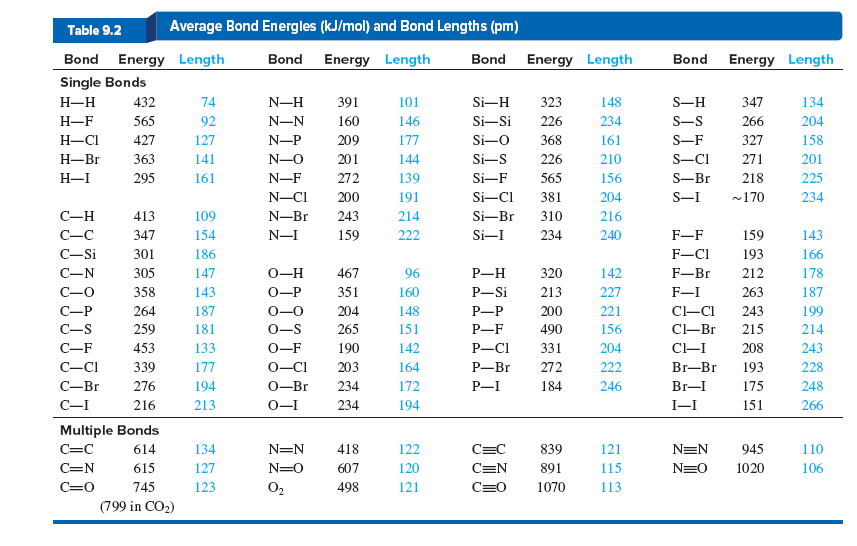# Problem: The average C—H bond energy in CH4 is 415 kJ/mol. Use following table and the following data to calculate the average C—H bond energy in ethane (C2H6; C—C bond), in ethene (C2H4; C=C bond), and in ethyne (C 2H2; C≡C bond):C2H6(g) + H2(g) ⟶ 2CH4(g)               ΔH°rxn =    −65.07 kJ/molC2H4(g) + 2H2(g) ⟶ 2CH4(g)             ΔH°rxn = − 202.21 kJ/molC2H2(g) + 3H2(g) ⟶ 2CH4(g)             ΔH°rxn =   −376.74 kJ/mol

###### FREE Expert Solution

We’re being asked to calculate the calculate the average C—H bond energy in ethane (C2H6; C—C bond), in ethene (C2H4; C=C bond), and in ethyne (C 2H2; C≡C bond).

To calculate the ΔH°rxn using bond energies, we’re going to use the following equation:

Let’s first figure out what kind of bonds and how many moles are present in each reactants and products because the given bond energies are per mole.

86% (143 ratings)###### Problem Details

The average C—H bond energy in CH4 is 415 kJ/mol. Use following table and the following data to calculate the average C—H bond energy in ethane (C2H6; C—C bond), in ethene (C2H4; C=C bond), and in ethyne (C 2H2; C≡C bond):

C2H6(g) + H2(g) ⟶ 2CH4(g)               ΔH°rxn =    −65.07 kJ/mol
C2H4(g) + 2H2(g) ⟶ 2CH4(g)             ΔH°rxn = − 202.21 kJ/mol
C2H2(g) + 3H2(g) ⟶ 2CH4(g)             ΔH°rxn =   −376.74 kJ/mol